# Formula for curvature of tensor product of connections

Suppose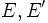$E,E'$ are vector bundles over a differential manifold$M$. Suppose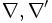$\nabla,\nabla'$ are connections on$E,E'$ respectively. Let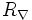$R_\nabla$ denote the Riemann curvature tensor of$\nabla$ and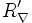$R_\nabla'$ denote that Riemann curvature tensor of$\nabla'$. Then if$E \otimes E'$ is the tensor product of vector bundles,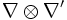$\nabla \otimes \nabla'$ is the tensor product of connections, and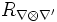$R_{\nabla \otimes \nabla'}$ is its Riemann curvature tensor, we have:$R_{\nabla \otimes \nabla'}(X,Y)(s \otimes s') = R_\nabla(X,Y)(s) \otimes s' + s \otimes R_\nabla(X,Y)s'$.